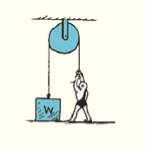×
Get Full Access to Conceptual Physics - 12 Edition - Chapter 2 - Problem 30e
Get Full Access to Conceptual Physics - 12 Edition - Chapter 2 - Problem 30e

×

# If the strong man in the exercise 1 exerts a downwardISBN: 9780321909107 29

## Solution for problem 30E Chapter 2

Conceptual Physics | 12th Edition

• Textbook Solutions
• 2901 Step-by-step solutions solved by professors and subject experts
• Get 24/7 help from StudySoup virtual teaching assistantsConceptual Physics | 12th Edition

4 5 1 271 Reviews
29
5
Problem 30E

If the strong man in the exercise 1 exerts a downward force of 800 N on the rope, how much upward force is exerted on the block? Exercise 1 For the pulley system shown, what is the upper limit of weight the strong man can lift?

Step-by-Step Solution:

Problem 30E

If the strong man in the exercise 1 exerts a downward force of 800 N on the rope, how much upward force is exerted on the block? Exercise 1 For the pulley system shown, what is the upper limit of weight the strong man can lift?

Step By Step Solution

Step 1 of 2Considering a massless string and a frictionless pulley, if a strongman exerts a downward force of 800N, an upward force of 800N is applied to the block.

Step 2 of 2

##### ISBN: 9780321909107

Unlock Textbook Solution